Next: 6.2.6 Vector-Matrix Notation Up: 6.2 Lateral Discretization of Previous: 6.2.4 Scaling of the

## 6.2.5 Truncation of the Fourier expansions

Note that up to now no approximations have been performed. We only inserted Fourier expansions into the Maxwell equations and thereby transformed the PDEs into ordinary ones. This means that the frequency domain formulation of (6.21) is totally equivalent to the spatial domain formulation of (6.5) provided, of course, that the EM field is laterally periodic. However, the rank of the ODE (6.21) is generally infinite, i.e., the dependence on the continuous spatial coordinates is transformed into an infinite number of Fourier coefficients. Only in case of homogeneous planar layers a finite number of Fourier coefficients suffices, i.e., those coefficients which are excited by the incident waves (cf. Appendix C). For a laterally constant permittivity, i.e.,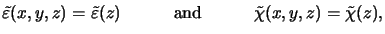(6.15)

the ODE coefficients of (6.22) have only diagonal entries since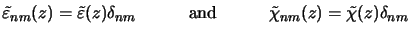(6.16)

holds in this case. EM scattering due to material inhomogeneity or the nonplanar material interfaces causes a coupling of all Fourier coefficients. The incident modes thus excite other, higher-order modes. The coupling degree is determined by the strength of the inhomogeneity or, in other words, by the number of the relevant Fourier coefficients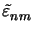(z) and(z) of the permittivity and its reciprocal, respectively. These basic considerations are helpful for the next step in the overall discretization procedure, namely the truncation of the Fourier expansions of the lateral field components.

The EM field inside the resist and wafer is excited by the diffraction orders collected by the projection lens (cf. 4.1). The number of plane waves traveling towards the resist is determined by the pupil function that is in fact a low-pass function (cf. (4.53) or (4.58)). Thus only low-order Fourier coefficients are directly excited by the incoming light, higher-order coefficients are indirectly stimulated by scattering effects. Hence we have to choose the truncation frequencies Nx and Ny higher than the cut-off frequencies of the projection lens. The exact values have to be chosen accordingly to catch enough coefficients so that scattering effects can be treated with sufficient precision. In the practical implementation the two cut-off frequencies Nx and Ny are input arguments to the simulator and it is the user's responsibility to choose them appropriately. Easy guidelines are given below, whereas an analysis of important implications due to the truncation can be found in Appendix D, where a lateral stepped permittivity as occurring at a nonplanar material interface is discussed.

The mathematical formulation of the truncation process writes as follows: Only coefficients symmetrically centered around the vertical incident ray n = m = 0 are considered, i.e., the index pair (n, m) must be an element of the set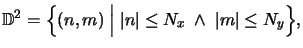(6.17)

whereas field coefficients not contained in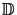2 are set to zero, i.e,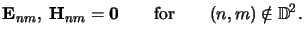(6.18)

This situation is graphically illustrated in Figure 6.3. Generally the real values of the neglected coefficients (n, m)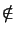2 will not vanish. Hence also the considered coefficients (n, m)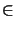2 are only calculated approximately since they also depend on the neglected ones due to the convolution-like relations in (6.19). Thus the approximations made throughout the truncation of the field expansions,(6.19)

entail two important implications:
1.
A low-pass filtering of the field.
2.
A low-pass filtering of the geometry.
The first is obvious and can directly be seen from the truncated series (6.27); the second, however, is somewhat more complicated. Namely, the medium is treated as if it were so smooth that no coupling to higher-order modes occurs (cf. (6.26)). Of course, this assumption is only true for small variations of the refractive index or, similarly, for smooth topography. Note that this limitation also applies to the second frequency domain method of the waveguide model (cf. Table 5.1) and is implicitly contained in any spectral method. The Fourier coefficients of the permittivity(z) and its reciprocal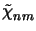(z), on the other hand, can be exactly computed. Because of the truncation of the field expansions and due to the convolution-like relations in (6.19) it suffices to calculate coefficients(z) and(z) up to an order of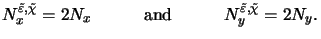(6.20)

Hence, roughly twice as many coefficients of the permittivity and its reciprocal as of the field are required. These relations are also sketched in Figure 6.3.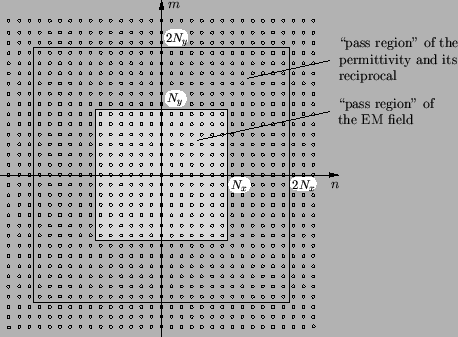The rank of the ODE system (6.21) is now finite and equals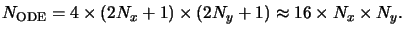(6.21)

The factor four is due to the four lateral field components, the cut-off frequencies are counted twice to account for positive'' as well as negative'' harmonics, and the additive one results from the zeroth-order harmonic.Next: 6.2.6 Vector-Matrix Notation Up: 6.2 Lateral Discretization of Previous: 6.2.4 Scaling of the
Heinrich Kirchauer, Institute for Microelectronics, TU Vienna
1998-04-17